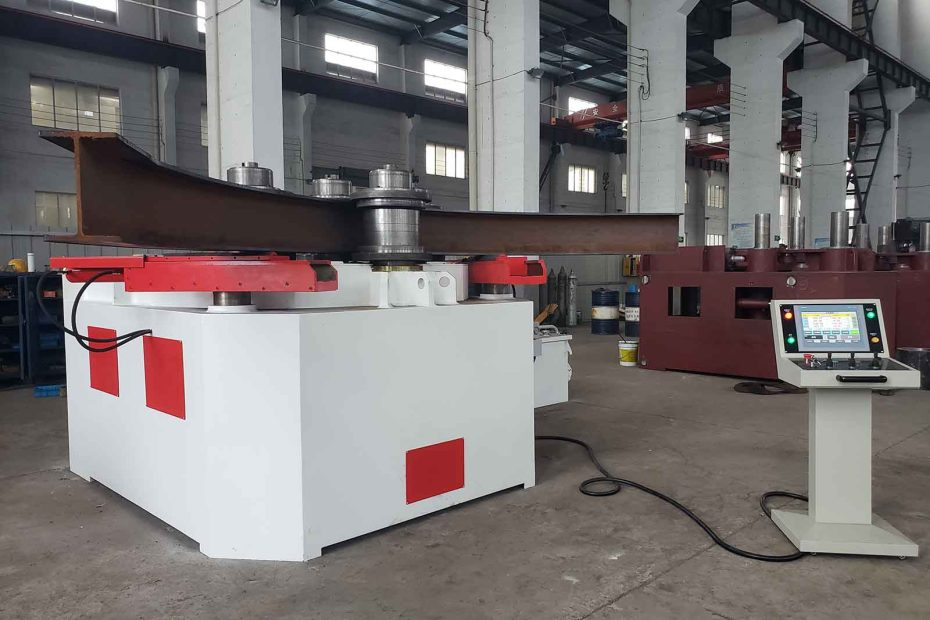# Beam Bending vs. Axial Load: How to Calculate Combined StressesIn structural engineering, beams often experience a combination of bending and axial load, which can result in complex stress conditions. Understanding how to calculate combined stresses is crucial for analyzing and designing beams that are subject to both bending moments and axial forces.

## Beam Bending and Axial Load: An Overview

Beam bending occurs when external loads cause the beam to deform in a curved shape. This deformation results in stresses within the beam, with tension on one side and compression on the other. Axial load, on the other hand, refers to the force acting along the longitudinal axis of the beam, causing either tension or compression throughout its length. When beams are subjected to both bending and axial load simultaneously, the resulting stresses can be more complex and require careful analysis.

## Calculating Combined Stresses

To calculate combined stresses in beams experiencing both bending and axial load, the following steps should be followed:

1. Determine the Bending Stress: The bending stress in a beam can be calculated using the formula:

σ_b = (M * y) / I

where σ_b is the bending stress, M is the bending moment, y is the distance from the neutral axis to the point of interest, and I is the moment of inertia.

1. Determine the Axial Stress: The axial stress in a beam can be calculated using the formula:

σ_a = F / A

where σ_a is the axial stress, F is the axial force, and A is the cross-sectional area of the beam.

1. Calculate the Combined Stress: The combined stress can be determined by summing the bending stress and the axial stress:

σ_combined = σ_b + σ_a

Note that the signs of the stresses should be taken into account, considering the tension-compression convention. Tension is considered positive, while compression is negative.

### Factors Affecting Combined Stresses

To better understand the factors influencing combined stresses, consider the following:

1. Magnitude of Bending Moment and Axial Force: Higher bending moments and axial forces result in increased stresses, potentially pushing the beam closer to its limit.
2. Beam Geometry: The shape and dimensions of the beam’s cross-section, such as depth and width, influence the moment of inertia and the distribution of stresses.
3. Eccentricity of Axial Load: When the axial load does not act through the centroid of the cross-section, it introduces additional bending moments, affecting the stress distribution.
4. Beam Material Properties: The material properties, such as yield strength and modulus of elasticity, play a crucial role in determining the beam’s ability to withstand combined stresses.

### Example of Combined Stress Calculation

Consider a beam subjected to a bending moment of 100 kNm and an axial force of 200 kN. The beam’s cross-sectional properties are as follows:

Moment of inertia (I) = 10,000 cm^4 Cross-sectional area (A) = 500 cm^2

Using the formulas mentioned earlier, the combined stress can be calculated as follows:

## Conclusion

In conclusion, understanding how to calculate combined stresses in beams experiencing both bending and axial load is crucial for designing safe and efficient structures. By considering the bending stress and axial stress separately and summing them appropriately, engineers can determine the combined stress. Factors such as the magnitude of bending moment and axial force, beam geometry, eccentricity of axial load, and material properties all influence the resulting combined stresses. Through careful analysis and design considerations, engineers can ensure that beams can withstand the complex stress conditions associated with combined loading, leading to structurally robust and reliable structures.